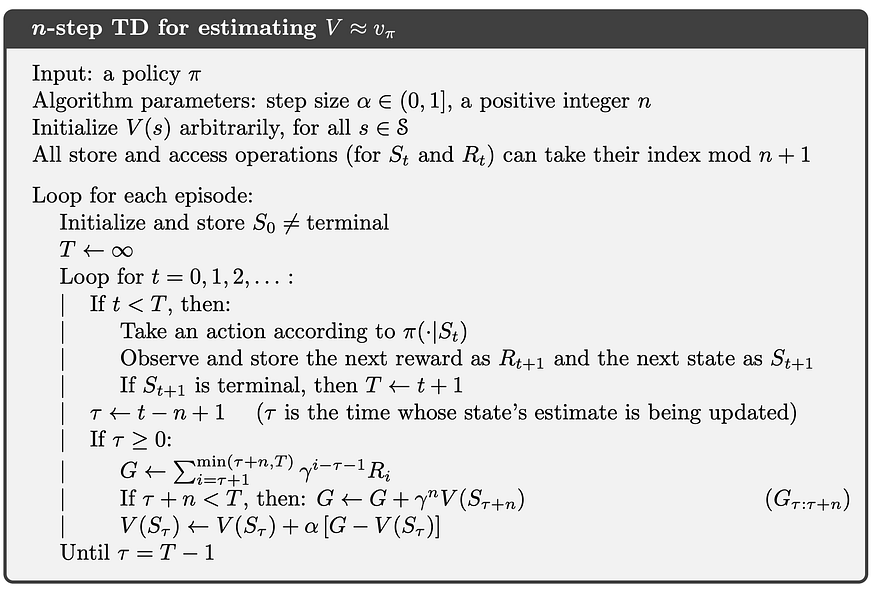# Why don't we bootstrap terminal state in n-step temporal difference prediction update equation?

In the algorithm below, when $$\tau + n \geq T$$, shouldn't the algorithm bootstrap with the value of the next state? For instance, when $$T=5, \tau=3, \& \; n=2$$, we don't bootstrap the sample return with $$V_{(\tau+n)}$$, i.e., $$V_5$$ or the terminal state.Also, on line 4, what do we mean by "can take their index mod $$n + 1$$"?